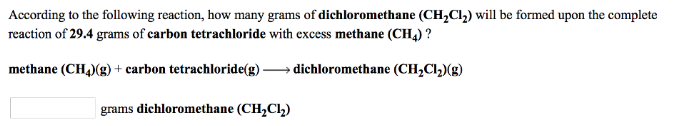# Problem: According to the following reaction, how many grams of dichloromethane (CH2Cl2) will be formed upon the complete reaction of 29.4 grams of carbon tetrachloride with excess methane (CH4) ? methane (CH4) (g) + carbon tetrachloride (g) → dichloromethane (CH2Cl2) (g)

###### FREE Expert Solution
97% (471 ratings)###### Problem Details

According to the following reaction, how many grams of dichloromethane (CH2Cl2) will be formed upon the complete reaction of 29.4 grams of carbon tetrachloride with excess methane (CH4) ?

methane (CH4) (g) + carbon tetrachloride (g) → dichloromethane (CH2Cl2) (g)